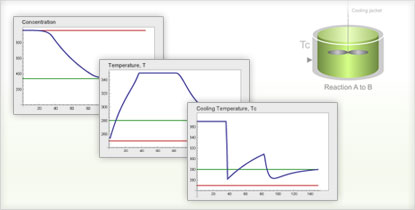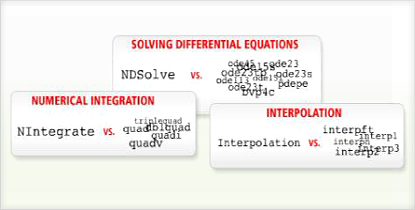# The Wolfram Solution for Chemical Engineering

Simulate your chemical processes with ready-to-deploy, fully interactive models using a combination of powerful computation, statistics and optimization, instant interactivity and built-in chemical data. One system, one integrated workflow.

Underlying the Wolfram chemical engineering solution is the world's most sophisticated differential equation solving with automatic algorithm selection, self-checking precision control and symbolic preprocessing–everything to get accurate results efficiently.

• The Wolfram Edge
• How Wolfram Compares
• Key Capabilities

Wolfram technologies include thousands of built-in functions that let you:

• Solve coupled nonlinear differential equations for chemical kinetic modeling and applications in transport phenomena
• Design and optimize unit operations such as distillation and adsorption processes in a chemical plant
• Develop thermodynamic models to predict vapor-liquid equilibria of non-ideal mixtures
• Compute Laplace transformations for process control applications
• Determine the numerical solution of transport equations, advanced heat and mass transfer problems and other transport phenomena applications
• Perform economic viability analysis for chemical plant design using built-in economic, financial, geographic and demographic data
• Improve system reliability
• Estimate the lifetime of mechanical assemblies and componentsFinding an optimal control signal for a continuously stirred tank reactor (CSTR) with Wolfram SystemModelerHighly optimized superfunctions for differential equation solving

• Instantly create dynamic interfaces that allow you to vary parameters and gain useful insights from data, a feature especially useful for reaction engineering/kinetics, process design and transport phenomena
Instant creation of interactive interfaces is not possible in other software
• Built-in, powerful statistical analysis and optimization routines for analyzing large amounts of data from experiments
Matlab requires extra-cost toolbox to perform statistical analysis
• Sophisticated typesetting for chemical and mathematical equations, standard word processing and interactive applications in a single document
Unique to Wolfram technologies
• Integrated automatic report generation to document tasks and instantly generate reports with graphics, text and code
Matlab requires purchase of extra toolbox; Sage, Octave and other freeware do not support automatic report generation
• Automated precision control and arbitrary-precision numerics produce highly accurate results
Fortran, Excel, Matlab and other systems relying on finite-precision numerics can create serious errors due to lack of precision
• Advanced string and pattern matching and sequence alignment capabilities for biochemical applications
Matlab requires purchase of toolbox
• Built-in parallel computing capabilities for solving computation- or data-intensive problems on multicore computers
Matlab requires purchase of extra toolbox for parallel computing; all other major software systems require extensive programming to parallelize processes
• Seamless integration of numerics, symbolics, interactive graphics and all other computational aspects in one document
Unique to Wolfram technologies